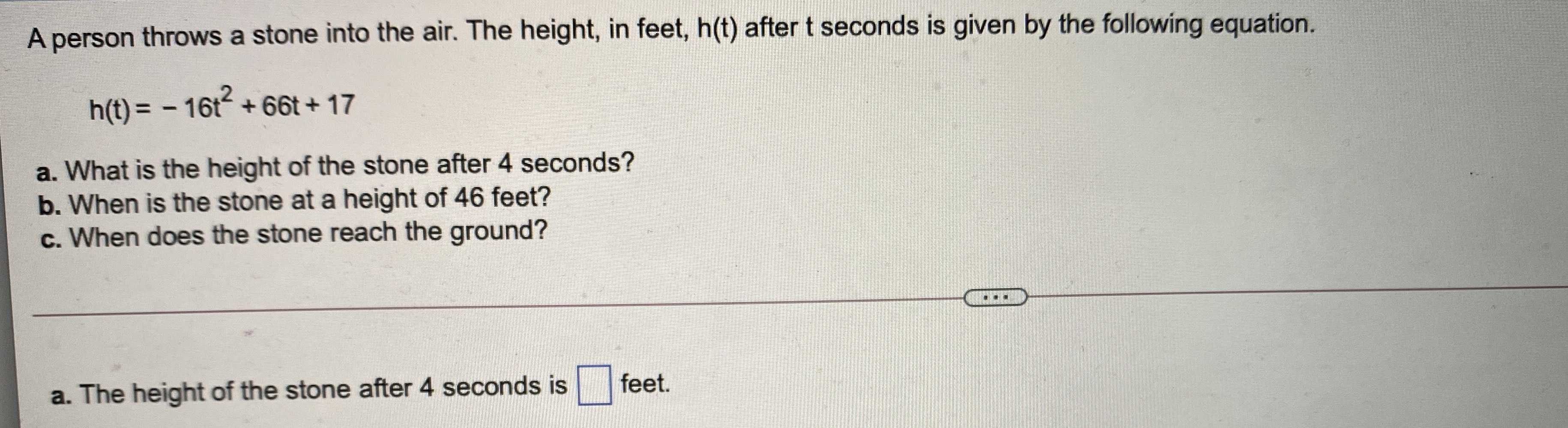### ¿Todavía tienes preguntas de matemáticas?

Pregunte a nuestros tutores expertos
Algebra
PreguntaA person throws a stone into the air. The height, in feet, $$h ( t )$$ after $$t$$ seconds is given by the following equation.

$$h ( t ) = - 16 t ^ { 2 } + 66 t + 17$$

a. What is the height of the stone after $$4$$ seconds?

b. When is the stone at a height of $$46$$ feet?

c. When does the stone reach the ground?